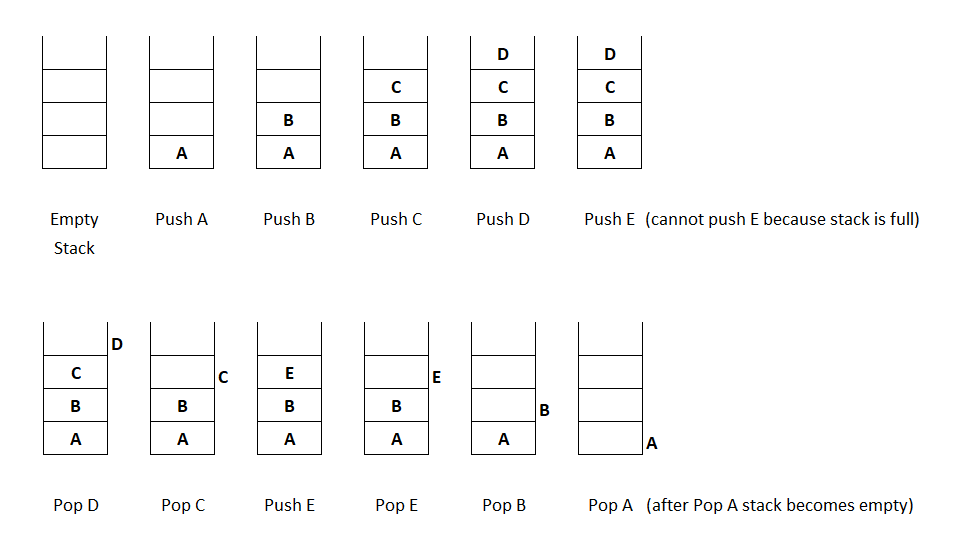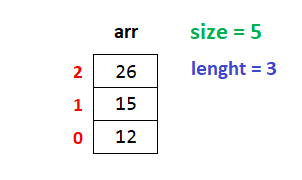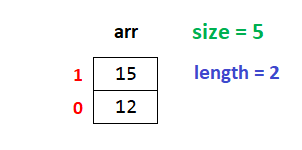# Stack in Python Programming

###### User-Defined Data Structures in Python

In this lesson, we will understand what is Stack in Python Programming and how to create them along with some examples.

## What is Stack in Python

A Stack in Python is a user-defined data structure in which we can insert or delete elements only at the top of the stack.

We can see the example of a stack in our daily life as the stack of books.In the above image, we can see that we can take a book only from the top. Similarly, we can keep one more book only at the top.## Operation on Stack

There are two operations possible on the stack.

• Push - When we add an element in the stack.
• Pop - When we delete an element from the stack.

To understand how the above operations work on a stack. See the example given below.From the above image, we can see that the elements added (push) last in the stack are deleted (pop) first from the stack.

The stack behaves in a last in, first out manner. It means that when an element is added to a stack, it becomes the last element unless we add more, and when we remove an element from the stack, the last added element is removed first.

So a stack is known as LIFO (Last In First Out) data structure.

## Implementation of Stack in Python

The stack in python programming can be implemented in two ways using:

• Array or List

In this lesson, we will see the implementation of a stack using an array. We will discuss how to implement stack using a singly linked list later in the subsequent lesson.

### Push Operation on Stack using Array

Suppose, we want to store 5 numbers in a stack. In that case the maximum size of the array will be 5.

For push operation on the stack first, we check if the array's length is equal to the size of the array. Then, we will display a message Stack is full. Else we will append the number in the array.

#### Example

``````from array import *
size = 5
arr = array('i',[])

if len(arr)==size:
print('Stack is full')
else:
n=int(input('Enter a number '))
arr.append(n)``````

If we insert three elements, say 12, 15, and 26, into the stack, the stack will look as shown in the image below.### Pop Operation on Stack using Array

For pop operation on the stack first, we check if the array's length is equal to 0. Then, we will display a message Stack is empty. Otherwise, we will remove the last number from the array using the pop() function.

#### Example

``````if len(arr)==0:
print('Stack is empty')
else:
print('Popped number = %d' %(arr.pop()))``````

If we delete the last element, 26, from the stack, the stack will look as shown in the image below.## Program of Stack using Array

Below is the complete program of the stack in python using an array of size 5.

#### Example

``````from array import *
import os

size = 5    # Maximum numbers to be stored in the array. We can increase the quantity by changing the value 5 with a new one
arr = array('i',[])     # An empty array for stack implementation

while 1:    # An infinite while loop
os.system('cls')    # Clear the console screen in Windows OS. For Linux and MacOS use os.system('clear')
print('1. Push')
print('2. Pop')
print('3. Display')

if ch==1:
# Check if the stack is full
if len(arr)==size:
print('Stack is full')
input('Press enter to continue...')     # Pause the loop so that the user can see the above message Stack is full
else:
# If stack is not full then append the number in the array
n=int(input('Enter a number '))
arr.append(n)

elif ch==2:
# Check if the stack is empty
if len(arr)==0:
print('Stack is empty')
input('Press enter to continue...')     # Pause the loop so that the user can see the above message Stack is empty
else:
# If stack is not empty then remove the last number from the array
print('Number Popped = %d' %(arr.pop()))
input('Press enter to continue...')     # Pause the loop so that the user can see the above message Number Popped = ?

elif ch==3:
# Check if the stack is empty
if len(arr)==0:
print('Stack is empty')
input('Press enter to continue...')     # Pause the loop so that the user can see the above message Stack is empty
else:
# if stack is not empty then display all the number starting from backside
for i in range(len(arr)-1,-1,-1):
print(arr[i])
input('Press enter to continue...')     # Pause the loop so that the user can see full stack on the screen
else:
print('Invalid Choice')
input('Press enter to continue...')     # Pause the loop so that the user can see the above message Invalid Choice``````